## Cramer's Rule

Given a set of linear equations(1)

consider the Determinant(2)

Now multiplyby, and use the property of Determinants that Multiplication by a constant is equivalent to Multiplication of each entry in a given row by that constant(3)

Another property of Determinants enables us to add a constant times any column to any column and obtain the same Determinant, so addtimes column 2 andtimes column 3 to column 1,(4)

If, then (4) reduces to, so the system has nondegenerate solutions (i.e., solutions other than (0, 0, 0)) only if(in which case there is a family of solutions). Ifand, the system has no unique solution. If insteadand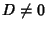, then solutions are given by(5)

and similarly for(6)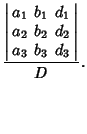(7)

This procedure can be generalized to a set ofequations so, given a system oflinear equations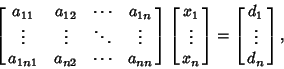(8)

let(9)

If, then nondegenerate solutions exist only if. Ifand, the system has no unique solution. Otherwise, compute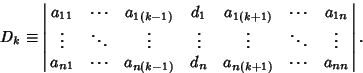(10)

Then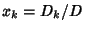for. In the 3-D case, the Vector analog of Cramer's rule is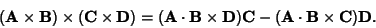(11)